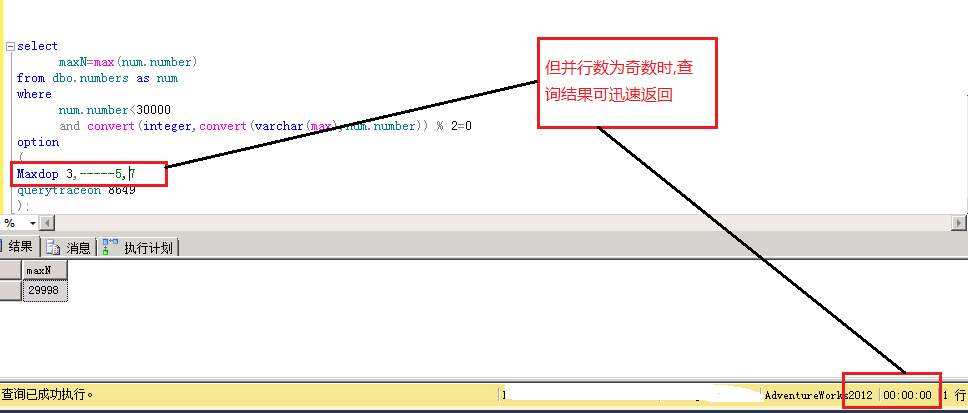# SQL Server并行死锁案例解析

并行死锁我们可以理解为SQL Server的”BUG”,即按照并行方式执行时遭遇特定异常使得执行无法继续.官方给的解决方式大多很简单,改为串行就可以了.但其中还是有值得我们深究的地方以便我们更好的利用并行.

注:由于案例涉及的并行知识点较多,缺乏相关知识的同学可以先预览一下我当时分享的PPT,如果有不清楚的地方也可以私信我. Inside Parallel Query

闲篇到此,上实例``````CREATE TABLE Numbers(  Number INT NOT NULL,  CONSTRAINT PK_Numbers     PRIMARY KEY CLUSTERED (Number)    WITH FILLFACTOR = 100)INSERT INTO NumbersSELECT  (a.Number * 256) + b.Number AS NumberFROM   (    SELECT number    FROM master..spt_values    WHERE       type = 'P'      AND number <= 255  ) a (Number),  (    SELECT number    FROM master..spt_values    WHERE       type = 'P'      AND number <= 255  ) b (Number)
``````

View Code``````set statistics time onselect    maxN=max(num.number)from dbo.numbers as numwhere    num.number<30000   and convert(integer,convert(varchar(max),num.number)) % 2=0option(Maxdop 3,-----5,7querytraceon 8649);
``````

View Code``````select    maxN=max(num.number)from dbo.numbers as numwhere    num.number<30000   and convert(integer,convert(varchar(max),num.number)) % 2=0option(Maxdop 4,-----2,6querytraceon 8649);
``````

View Code图1-1图1-3

1访问基表数据时用的是聚集索引扫描,但扫描方式是backward,而SQL server中只有forward scan可以并行扫描,backward只能串行扫描图2-2